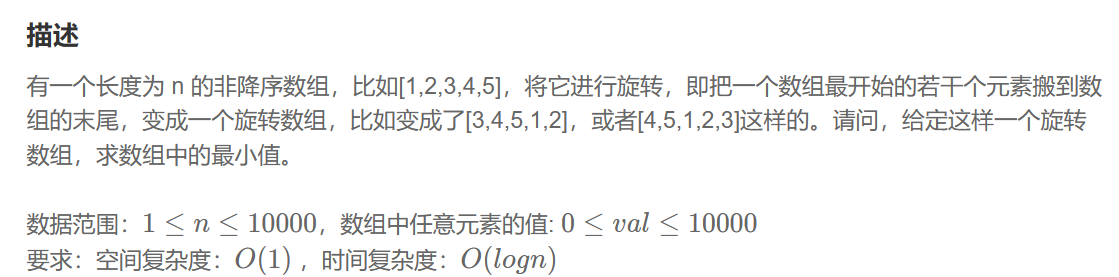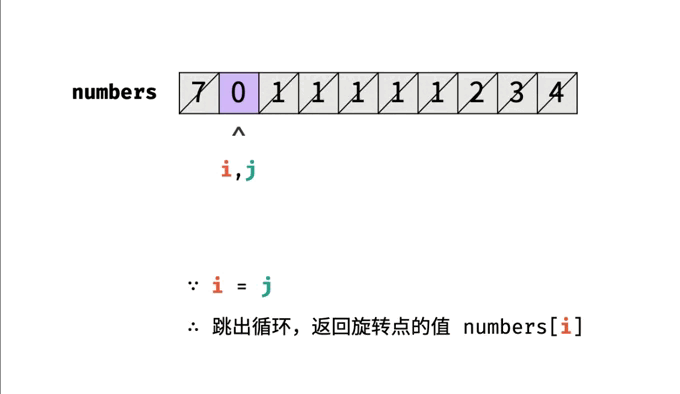# 【剑指offer】旋转数组的最小数字• 👑专栏内容：剑指offer
• ⛪个人主页：子夜的星的主页
• 💕座右铭：前路未远，步履不停

• 一、题目描述
• 1、题目
• 2、示例
• 示例1
• 示例2
• 二、题目分析
• 1、暴力法
• 2、二分法
• 三、代码汇总
• 1、暴力法
• 2、二分法

# 一、题目描述

## 1、题目# 二、题目分析

## 1、暴力法

``````class Solution {
public:
int minArray(vector<int>& numbers) {
int n = numbers.size();		//（1）
int min = numbers;		//（2）
for(int i = 1;i<n;i++) 		//（3）
{
if(numbers[i] < numbers[i-1])	//（4）
{
min = numbers[i];
break;			//（5）
}
}
return min;
}
};
``````

（1）获取旋转数组的长度

（2）让旋转数组中第一个元素为最小值

（3）从第二个元素开始遍历旋转数组

（4）如果当前元素比前一个元素小，证明引出现了递减，那么当前元素就是旋转数组的最小元素

（5）找到了最小元素，跳出循环

## 2、二分法``````class Solution {
public:
int minArray(vector<int>& numbers) {
int n = numbers.size();
int left = 0,right = n-1;
//二分查找
while(left<right)
{
int mid = (left + right)/2;
if(numbers[mid]>numbers[right])
left = mid + 1;
else if (numbers[mid]<numbers[right])
right = mid;
else
right --;
}
return numbers[left];
}
};
``````

# 三、代码汇总

## 1、暴力法

``````class Solution {
public:
int minArray(vector<int>& numbers) {
int n = numbers.size();
int min = numbers;
for(int i = 1;i<n;i++)
{
if(numbers[i] < numbers[i-1])
{
min = numbers[i];
break;
}
}
return min;
}
};
``````

## 2、二分法

``````class Solution {
public:
int minArray(vector<int>& numbers) {
int n = numbers.size();
int left = 0,right = n-1;
//二分查找
while(left<right)
{
int mid = (left + right)/2;
if(numbers[mid]>numbers[right])
left = mid + 1;
else if (numbers[mid]<numbers[right])
right = mid;
else
right --;
}
return numbers[left];
}
};
``````